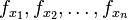Continuous partials implies differentiable

Statement

Suppose$f$ is a real-valued function of$n$ variables$x_1,x_2,\dots,x_n$. Suppose that$(a_1,a_2,\dots,a_n)$ is a point in the domain of$f$ such that the partial derivatives$f_{x_1}, f_{x_2},\dots,f_{x_n}$ exist and are continuous at and around the point$(a_1,a_2,\dots,a_n)$ (i.e., they all exist and are continuous in an open ball containing$(a_1,a_2,\dots,a_n)$). Then, the gradient vector of$f$ exists at$(a_1,a_2,\dots,a_n)$ and is given by (as per relation between gradient vector and partial derivatives):$(\nabla f)(a_1,a_2,\dots,a_n) = \langle f_{x_1}(a_1,a_2,\dots,a_n), f_{x_2}(a_1,a_2,\dots,a_n), \dots, f_{x_n}(a_1,a_2,\dots,a_n) \rangle$

Note that we say a function of multiple variables is differentiable if the gradient vector exists, hence this result can be restated as continuous partials implies differentiable.

Significance

In general, computing partial derivatives is easy, but computing the gradient vector from first principles is hard. Fortunately, we have a result, called the relation between gradient vector and partial derivatives, that says that the coordinates of the gradient vector, wherever it does exist, are simply the partial derivatives. Explicitly, it says that:$(\nabla f)(a_1,a_2,\dots,a_n) = \langle f_{x_1}(a_1,a_2,\dots,a_n), f_{x_2}(a_1,a_2,\dots,a_n), \dots, f_{x_n}(a_1,a_2,\dots,a_n) \rangle$

The problem with that result is that it holds conditional to the existence of the gradient vector. In fact, existence of partial derivatives not implies differentiable. Thus, after computing the partial derivatives, we know what the gradient vector should be if it exists, but we still aren't sure whether it exists.

That is where the result of this page comes in useful. It allows us to use the continuity of the partial derivatives, that we have already computed, to deduce the existence of the gradient vector, and hence also compute an expression for it.

Related facts

Converse

The converse is not true: the existence of the gradient vector does not imply the continuity of the partial derivatives, and it does not even imply the existence of partial derivatives around the point. In fact, we can construct counter-examples with functions of one variable. See differentiable not implies continuously differentiable.